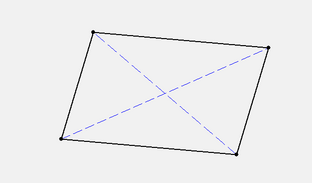## Introduction

Given four points $A,B,C$, and $D$ in the plane, denote $ABCD$ by the quadrilateral formed by joining $A$ to $B$, $B$ to $C$, $C$ to $D$, and $D$ to $A$. When is such a figure a parallelogram? By definition, when opposite sides are parallel and equal length. In other words, when

 $B-A=C-D$ $A+C=B+D$ $\frac{A+C}{2}=\frac{B+D}{2}$.

This proves the

### Discussion

Which of expressions above are independent of the origin?

The first, $B-A$, is because it is a displacement vector: it represents the change from one point to another in the plane. Conceptually, displacements are not the same thing as locations, because a location is defined relative to a fixed origin and a displacement is defined relative to the two points.

The second expression $A+C$ is not independent of the origin, but the third, $\frac{A+C}{2}$ is. Superficially, the difference appears to be only that we divided the equation by two. However, let’s rewrite the third expression as $\frac{1}{2}A+frac{1}{2}C$. The important thing to notice is that the coefficients $(\frac{1}{2},\frac{1}{2})$ add up to 1, while the coefficients of $A+C$ do not. What this means is that the point a $\frac{1}{2}A+\frac{1}{2}C$ lies on the line through $A$ and $C$; we don’t need a fixed origin because the point is referenced relative to $A$ and $C$.Click image to download quad-1.seg and open it in KSEG.

## Notation

Some notation we’ll use: the line joining two points $A$ and $B$ can be written

 $\ell_{AB}={X\ |\ X=aA+bB, a+b=1}$.

We’ll also write $(AB)$ for $\ell_{AB}$ because it can be typed in an email and it’s shorter. A few more: write the intersection of two lines $\ell, m$ as $(\ell m)$; and $(A\ell)$ will be short for $A\in\ell$, $A$ is on $\ell$, or $\ell$ goes thru $A$.

Question.

What should $(ABC)$ mean?

Associate $(ABC) = A(BC)$, and we read that $A$ is a point on the line $(BC)$ through these points. Our textbook by Tondeur would write this as $A \in \ell_{BC}$, which is hard to write in an email. Thus the three points are collinear. Note that we wouldn’t write $(ABC)$ if we didn’t mean to say that all three points are distinct.

Note that we can use the commutative and associative rules and continue to say the same thing. For example, $(ABC)=B(CA)$ still says that the three points lie on the same line.

Question.

What should $not(ABC)$ mean?

The not symbol means the negation of the statement. So, since $A,B,C$ are distinct, and not collinear, they must be the vertices of a triangle.
We prefer to write this so $\triangle ABC$.